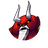## Getting the lowest value excluding 0 document.SUBSCRIPTION_OPTIONS = { "thing": "topic", "subscribed": false, "url": "subscribe", "icon": { "css": "fa fa-envelope-o" } };

Ringo
2019-01-20
2019-01-24
•Ringo - 2019-01-20

I've really struggled to find a way to do this but I cant seem to get it to work.

I want to get the minimum value from X amount of INT variables but not if its 0.

Theese are the once Ive tryed (and countless variations with <> 0 and so on):

Lets say the values are Var1 = 3, Var 2 = 5, Var3 = 0 and Var4 = 10

NewVariable := MIN(Var1, Var2, Var3, Var4);

This gives me the value 0.

NewVariable := LIMIT(1,(MIN(Var1, Var2, Var3, Var4)),15);

This gives me the value 1.

The value i really want is 3, which is the lowest value that is NOT 0.

•ndzied1 - 2019-01-21

A little brute force but you could do something like this.

Define a variable for the minimum value
MinVal: int := 32000;

```IF Var1 <> 0 THEN
MinVal := Min(MinVal,Var1);
END_IF
IF Var2 <> 0 THEN
MinVal := Min(MinVal,Var2);
END_IF
IF Var3 <> 0 THEN
MinVal := Min(MinVal,Var3);
END_IF
IF Var4 <> 0 THEN
MinVal := Min(MinVal,Var4);
END_IF
IF Var5 <> 0 THEN
MinVal := Min(MinVal,Var5);
END_IF
```

If you put the values in an array, then you could loop through it in a FOR loop.

•Ringo - 2019-01-21

Thanks, that almost worked. But I want the MinValue to be at 0 value until 1 or more of Val1-5 goes over 0, then i want MinValue to have that value...

Maybe I should start to look for a diffrent solution.

Iam making an elevator for school, and Ive added a second elevatorcar and I want the it to go down
to the lowest floor as soon as 1 of 5 variables becomes active. If the MinValue is 0 the elevator stops.

•dFx - 2019-01-21
```MinValueNonZero := 0;
FOR index:=1 TO 4
IF ElevatorBtn[index] > 0 THEN
IF MinValueNonZero = 0 THEN
MinValueNonZero := ElevatorBtn[index];
ELSE
IF MinValueNonZero > ElevatorBtn[index] THEN
MinValueNonZero := ElevatorBtn[index];
END_IF;
END_IF;
END_IF;
END_FOR;
```

EDIT 2019/01/24 : Correct fix for first value

•Ringo - 2019-01-23

dFx hat geschrieben:

```MinValueNonZero := 0;
FOR index:=1 TO 4
IF ElevatorBtn[index] > 0 THEN
IF MinValueNonZero > ElevatorBtn[index] THEN
MinValueNonZero := ElevatorBtn[index];
END_IF;
END_IF;
END_FOR;
```

Genius

I solved it by using a whole bunch of IF statments but I will change that för this. Thanks!

•dFx - 2019-01-24

Edited my code